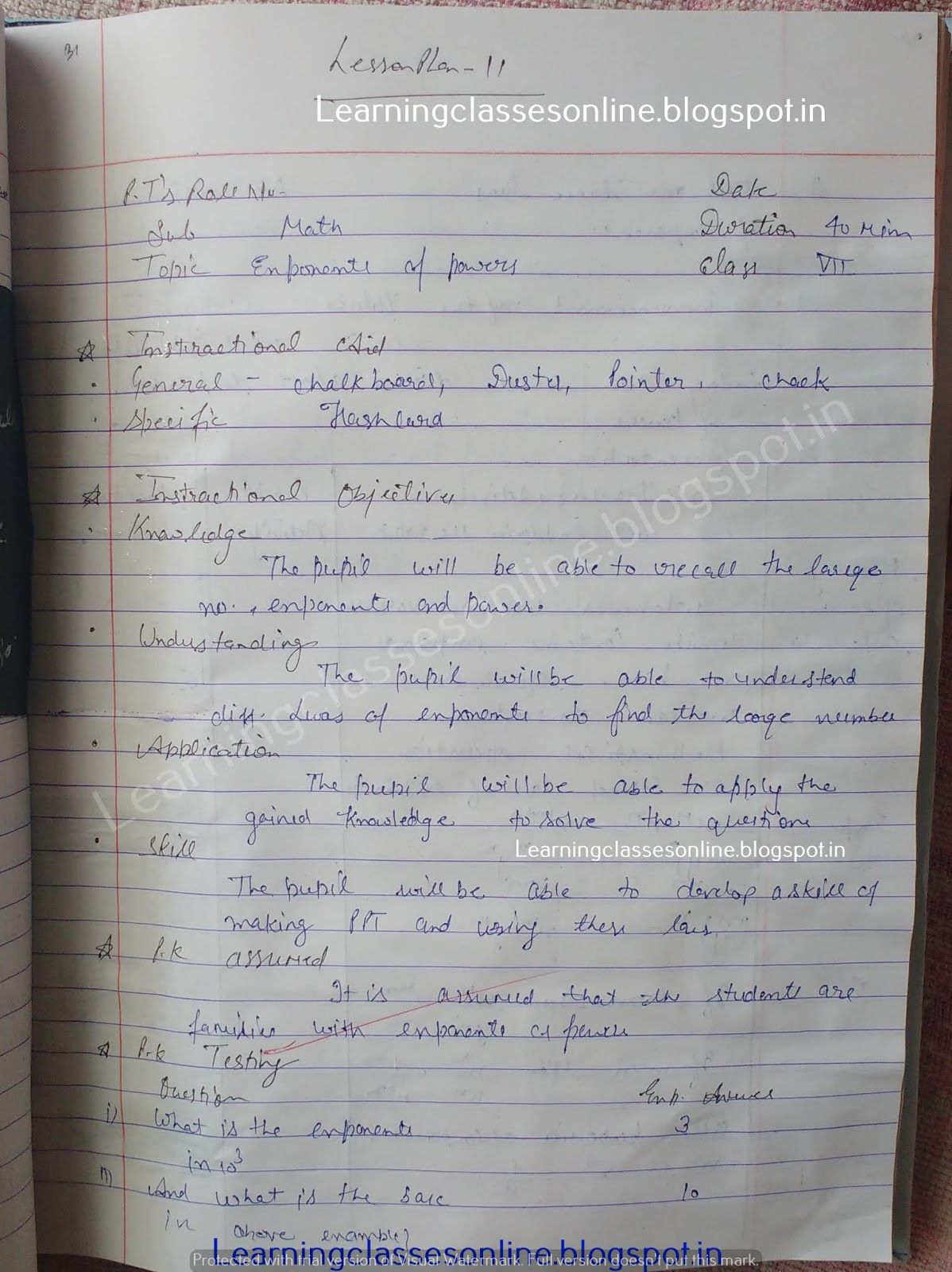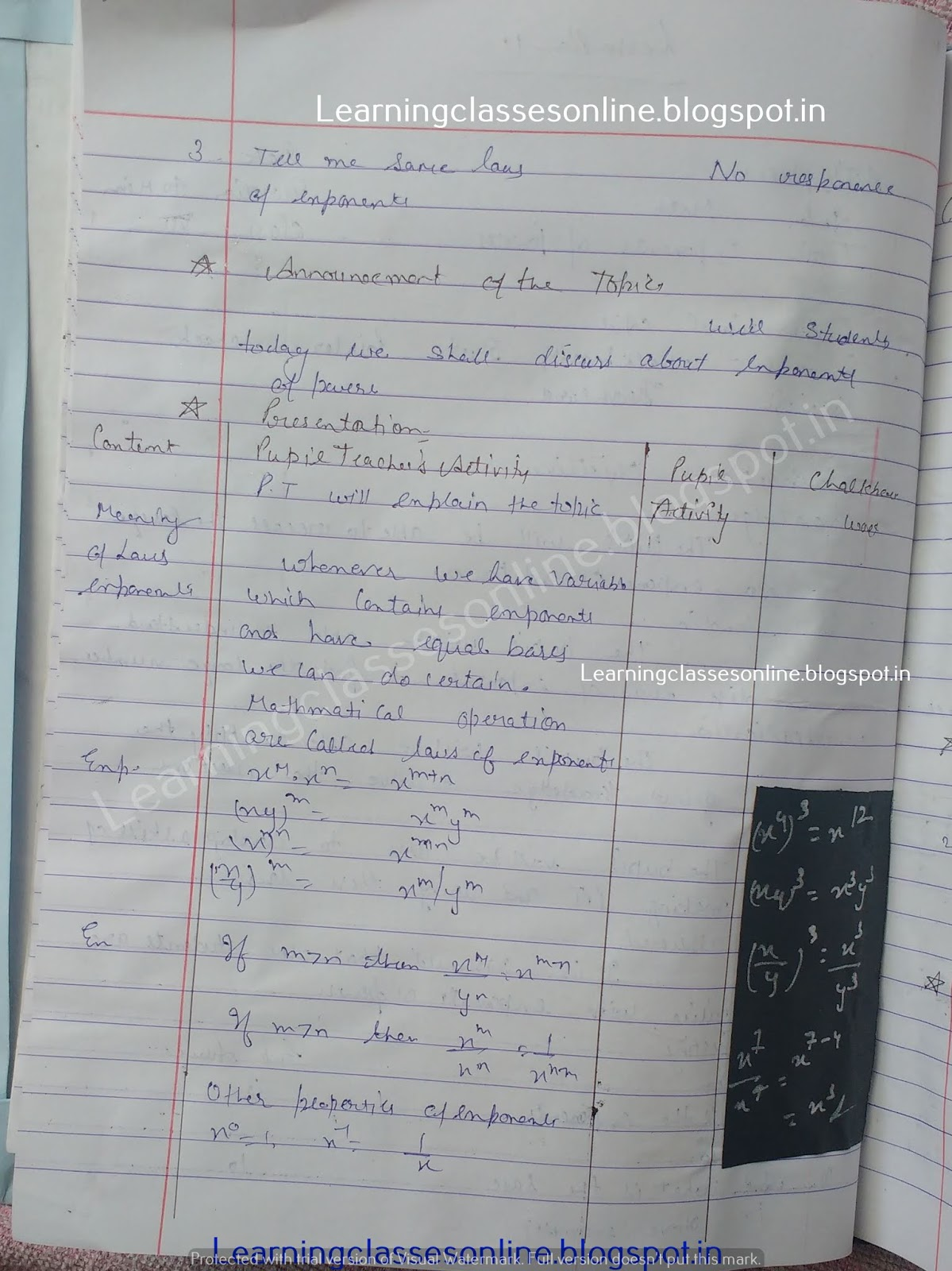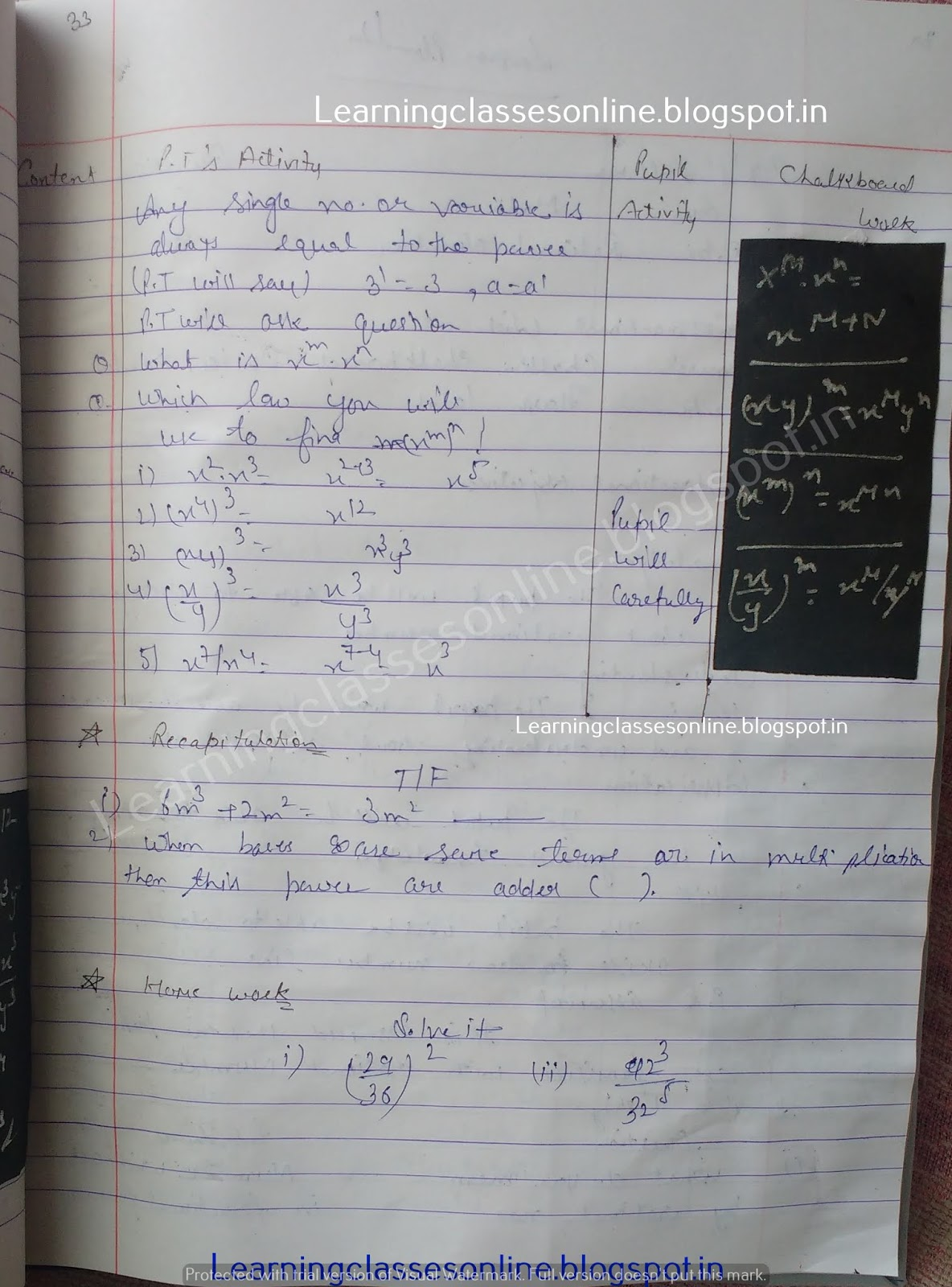Math teaching lesson plan on exponents of powers -->

# Math teaching lesson plan on exponents of powers

sample and model Math teaching lesson plan on exponents of powers for mathematics teachers

Note: The Mathematics Lesson Plan given below is just an example. You can change the Name, Class, Course, Date, Duration, etc. according to your needs.#### Related Posts

1.